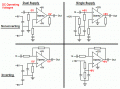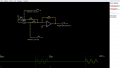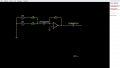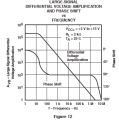# Calculating Coupling and Decoupling Capacitors

#### Yeye

Joined Nov 12, 2019
47
Hey guys, I want to build a circuit that sums up a Stereo Signal ( Aux / Phone Voltage ) to a Mono one and afterwards Filter it. I know, how my circuit should look and since i want to power it with a Single Supply, i have to use a coupling capacitor at the input and one at the output of each opamp stage, right?
Audioguru again
sent me this picture in my last Post:I will be using the method at the bottom right Corner, since my Summing Amplifier circuit looks like that:I also added a Line out, which shouldnt really be a Problem at all, right?
So if i now use the Method, Audioguru sent me a picture of, I have to use a coupling capacitors between the 10k Ohm resistors behind the Signal channels and the inverting opamp input, right? The opamp i want to use is the TL072 . It has got an extremely high Input impedance in the height of Gigaohms or even Tera ohms, if i remember correctly, so i dont have to look at this when choosing the Capacitor.
I saw a formula at a website that describes, how to choose that capacitor, but i have to know the input impedance of the Circuit. The formula then is:
C=1/2_3.14_f *Xc ( I dont know what "_" should mean, please explain if you know )

What is the input Impedance of that Circuit, i want to design?That's just for the first, i will come to my other Questions after i understood This.
Greetings

#### dl324

Joined Mar 30, 2015
13,491
The formula then is:
C=1/2_3.14_f *Xc ( I dont know what "_" should mean, please explain if you know )
They mean multiply and parentheses should be around 2*pi*f*Xc.

#### DickCappels

Joined Aug 21, 2008
7,953

#### Yeye

Joined Nov 12, 2019
47
Yes, i know that Formula. Whats the input impedance of my circuit tho?
Thank you as always

#### KeepItSimpleStupid

Joined Mar 4, 2014
5,090
Look here: https://en.wikipedia.org/wiki/Line_level for a definition of line input and output impeadances.

Basically, you have consumer and professional levels. it's hard to find a pre-amp with 600 ohms in and 600 ohms out.
professional equipment is likely to be differential in, differential out and use an XLR connector.

#### Yeye

Joined Nov 12, 2019
47
0 ohms at the right, because the opamp draws no current in theory, right? Since the - input is a virtual ground, no current flows from it to the output, but the other way around it does. Is that correct?
The voltage sources are the channels of my Smartphones aux 3.5mm audio Jack out.

So if i want to calculate the Capacitor value, i have to know Zin of one channel and then take it Half (2 channels in parallel?), to add 5kOhms to it and then I know the impedance to calculate the capacitor value with. I use that Formula DickCappels sent me and that should be it.

The output capacitor is seperated, because the opamp also acts as a Buffer right?
Then i have to know the output impedance of my opamp and the impedance of the following circuit, right?
Thank you

#### DickCappels

Joined Aug 21, 2008
7,953
Right, correct, right.

The output impedance of your opamp is the feedback resistance divided by the opamp's open loop gain at the frequency of interest (highest frequency of interest). Actually its the open loop again +1 but you can read a Bode plot with so much accuracy. Remember to take the input impedance of the next stage into account.

Here's the plot for the TL07X series.#### Yeye

Joined Nov 12, 2019
47
Okay great, thank you!
Now, lets get to my text question:
how to calculate a decoupling capacitor?
I cant actually measure noise, but i know theres a lot of it, since my opamp output is noisy. My Power supply is a 24V Switch Mode psu.
Thank you as always

#### KeepItSimpleStupid

Joined Mar 4, 2014
5,090
0 ohms at the right, because the opamp draws no current in theory, right? Since the - input is a virtual ground, no current flows from it to the output, but the other way around it does. Is that correct?
this is probably messed up.

An ideal voltage source has 0 output Z and infinate input Z.

The OP-amp itself draws no current. Actually it's really tiny. pA or Femto-amps.

#### DickCappels

Joined Aug 21, 2008
7,953
Oddly the TL072 datasheet does not mention decoupling capacitors, otherwise I would just suggest following the recommendation in the datasheet. In this case (and I am designing with a TL072 this week) I use small electrolytic capacitors from the + and - supply pins to ground just as a safe practice -I always dislike reworking boards.

In the case where you can see noise on the output that you can correlate with noise on the power supply pins you can look at the common mode rejection ratio for the part and then design a filter network to give the desired attenuation. Sometimes this even gives the expected result!

#### Audioguru again

Joined Oct 21, 2019
3,821
Your schematic has the + input at ground so the supply must be dual polarity. For your single +24V supply then the + input must use a filtered voltage divider like I showed on my sketches about opamps.

#### MrAl

Joined Jun 17, 2014
8,545
Hey guys, I want to build a circuit that sums up a Stereo Signal ( Aux / Phone Voltage ) to a Mono one and afterwards Filter it. I know, how my circuit should look and since i want to power it with a Single Supply, i have to use a coupling capacitor at the input and one at the output of each opamp stage, right?
Audioguru again
sent me this picture in my last Post:
View attachment 193345
I will be using the method at the bottom right Corner, since my Summing Amplifier circuit looks like that:
View attachment 193346
I also added a Line out, which shouldnt really be a Problem at all, right?
So if i now use the Method, Audioguru sent me a picture of, I have to use a coupling capacitors between the 10k Ohm resistors behind the Signal channels and the inverting opamp input, right? The opamp i want to use is the TL072 . It has got an extremely high Input impedance in the height of Gigaohms or even Tera ohms, if i remember correctly, so i dont have to look at this when choosing the Capacitor.
I saw a formula at a website that describes, how to choose that capacitor, but i have to know the input impedance of the Circuit. The formula then is:
C=1/2_3.14_f *Xc ( I dont know what "_" should mean, please explain if you know )

What is the input Impedance of that Circuit, i want to design?
View attachment 193348
That's just for the first, i will come to my other Questions after i understood This.
Greetings
Hello,

To calculate a coupling capacitor you try to get a value that will pass all or most of the frequencies you need to pass so they get to the output without too much attenuation.

A common measure of this is the 3db down frequency. That is the frequency 'f' where the amplitude is about 71 percent of the maximum (the max is at infinite frequency).
However, you may not feel that 71 percent is quite good enough and it is hard to judge from that what you will get with the lowest frequency you want to pass if it has to be higher than 71 percent.

The typical 3db down frequency set point is calculated as:
f=1/(2*pi*R*C)

however you can calculate the 89 percent amplitude point just as easy:
f=1/(pi*R*C)

and that is the frequency where the amplitude is 89.4 percent of the maximum amplitude.
You can solve that for 'C' if you already know 'R' and you know the frequency 'f' you want to pass with at least 89 percent of the amplitude you get at higher frequencies.

The 95 percent amplitude frequency is also easy to calculate:
f=1.5/(pi*R*C)

That's the frequency where the amplitude is 94.9 percent of the maximum amplitude.

The three formulas are easy to remember.
f(71%)=0.5/(pi*R*C) {also called the 3db down formula}
f(89%)=1/(pi*R*C) {1db down formula}
f(95%)=1.5/(pi*R*C) {0.45db down formula nearly 0.5db down}

and note the numerator of each formula increments by 0.5 to get to the next formula starting with 0.5 in the 3db down formula and they all have pi*R*C in the denominator.

The value of R is found from the equivalent series R of the circuit.
For two 10k resistors in parallel that means R=5k.
For two 10k resistors in series, that means R=20k.

Last edited:

#### Audioguru again

Joined Oct 21, 2019
3,821
Your mixer opamp has its + input grounded, instead of biased, so it must use a positive and negative power supply.

#### MrAl

Joined Jun 17, 2014
8,545
Your mixer opamp has its + input grounded, instead of biased, so it must use a positive and negative power supply.

#### Audioguru again

Joined Oct 21, 2019
3,821
Maybe I said that in another forum to another person?

#### MrAl

Joined Jun 17, 2014
8,545
Maybe I said that in another forum to another person?

#### Audioguru again

Joined Oct 21, 2019
3,821
Since my computer died a few days ago I am tired of scroling and typing these threads on my phone.

#### MrAl

Joined Jun 17, 2014
8,545
Since my computer died a few days ago I am tired of scroling and typing these threads on my phone.
Ooh sorry to hear that. Maybe you can get a keyboard for your phone.